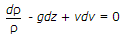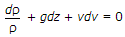# Civil Engineering - Hydraulics

### Exercise :: Hydraulics - Section 1

31.

A pipe of 0.1 m2 cross sectional area suddenly enlarges to 0.3 m2 cross-sectional area. If the discharge of the pipe is 0.3 m3 /sec, the head loss is

 A. 2/g m of water B. g/2 m of water C. 1g m of water D. g m of water.

Answer: Option A

Explanation:

No answer description available for this question. Let us discuss.

32.

Maximum efficiency of transmission of power through a pipe, is

 A. 25% B. 33.3% C. 50% D. 66.67%.

Answer: Option D

Explanation:

No answer description available for this question. Let us discuss.

33.

An ideal flow of a liquid obeys

 A. Continuity equation B. Newton's law of viscosity C. Newton's second law of motion D. dynamic viscosity law,

Answer: Option A

Explanation:

No answer description available for this question. Let us discuss.

34.

Euler's equation for motion of liquids, is given by

 A.B.C.D. ρdp + gdz + vdv = 0

Answer: Option C

Explanation:

No answer description available for this question. Let us discuss.

35.

Reynold number is the ratio of initial force and

 A. viscosity B. elasticity C. gravitational force D. surface tension.

Answer: Option A

Explanation:

No answer description available for this question. Let us discuss.

#### Current Affairs 2021

Interview Questions and Answers